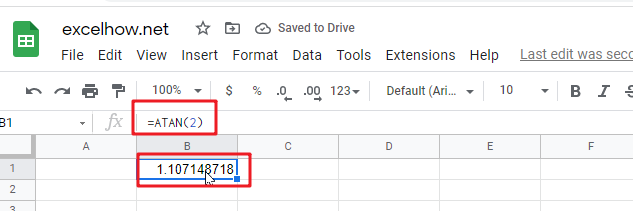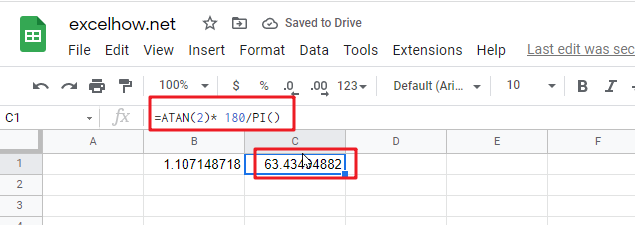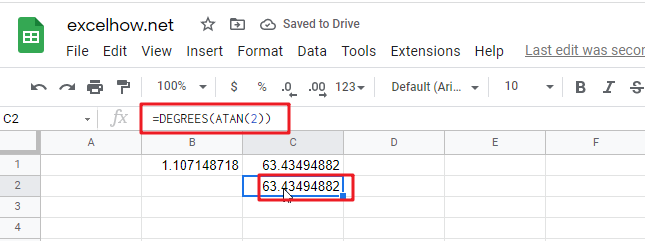This post will guide you how to use Google Sheets DEGREES function with syntax and examples.

## 1. Google Sheets DEGREES Function Description

The Google Sheets DEGREES function converts radians into degrees. And it will return a numeric value. For examples, if you pass the PI() function into the DEGREES function as its argument, and it will return 180.

The purpose of this function is used to convert radians to degrees in google sheet and it’s returned value is the degrees.

The DEGREES function is a build-in function in Google Sheets and it is categorized as a MATH function.

## 2. Google Sheets DEGREES Function Syntax

The syntax of the DEGREES function is as below:

=DEGREES (angle)

Where the DEGREES function argument is:

• angle -This is a required argument. The angle in radians that you want to convert to degrees.

## 3. Google Sheets DEGREES Function Examples

The below examples will show you how to use google sheets DEGREES Function to convert radians into degrees.

1# get the degrees of pi radians, enter the following formula in Cell B1.

``=DEGREES (PI())``

2# get the degrees of 1.25 radians, enter the following formula in Cell B2.

``=DEGREES (1.25)``

This post will guide you how to use Google Sheets ATAN function with syntax and examples.

## Description

The Google Sheets ATAN function returns the arctangent of a number. So this function can be used to calculates the arctangent of a supplied number. The returned angle is given in radians in the range –pi/2 to pi/2. Or the returned value is a numeric value.

The purpose of ATAN function is to get arctangent of a given number in google sheets.

The ATAN function is a build-in function in Google Sheets and it is categorized as a MATH function.

## Syntax

The syntax of the ATAN function is as below:

= ATAN (number)

Where the ATAN function arguments is:

• number -This is a required argument. The tangent of the angle that you want to get.

Note:

• If you want to express the arctangent in degrees, you just need to multiply the result by 180/PI() or you can use the DEGREES function.
• If the supplied number is not a numeric value, the ATAN function will get the #VALUE！ Error。

## Google Sheets ATAN Function Examples

The below examples will show you how to use google sheets ATAN Function to return the arctangent for a given number.

1# get the arctangent of 2 in radians, pi/4, enter the following formula in Cell B1.

`=ATAN(2)`The returned result is 1.107149.

2# get the arctangent of 2 in degrees, using the following formula in Cell B2.

`=ATAN(2)* 180/PI()`This formula will return the value 63.43495.

Or you can use the below formula to achieve the same result:

`=DEGREES(ATAN(2))`